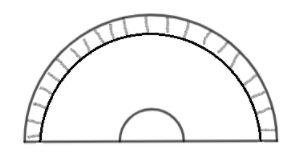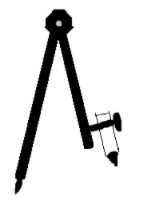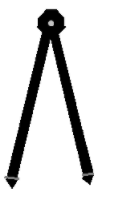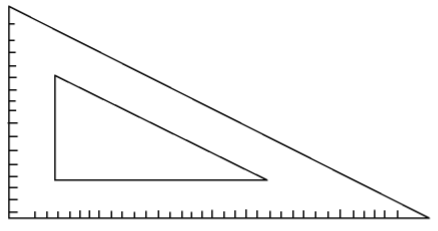Courses
Courses for Kids
Free study material
Offline Centres
MoreLast updated date: 05th Dec 2023
Total views: 279.6k
Views today: 5.79k

# The instrument in the geometry box having the shape of a triangle is called a(a)Protractor(b)Compasses(c)Divider(d)Set-squareVerified
279.6k+ views
Hint: We are required to identify the instruments we use in mathematics commonly found in a geometry box. We need to be aware of the basic instruments we use on a regular basis to help us draw better figures in mathematics than free hands. Here, four options are provided that are the names of four different instruments. We should know in advance what the geometry of each instrument is so that we are able to pick the correct option.

Complete step-by-step solution:
We first look at option (a). A Protractor is an angle measuring instrument. Say, we want to draw an angle of $36^{\circ}$. For that we need to first draw a line on which the angle will be made and then after using a protractor we can measure the angle in order to put the correct point so that we make the angle of accurate degree. A protractor has a shape like that of the capital alphabet D.So, option (a) would be wrong. Since, the shape of a protractor is of a semicircle.
Now, we look at option (b). A compass is an instrument used to draw circles. We simply put a pencil on one end of the compass and keeping the other end as centre we can easily draw the circle of required radius. A compass is a 3D structure that has two long metal plates joined together with one edge pointed and the other has a pencil holder.So, this option would also be discarded since it does not resemble the shape of a triangle.
We now look at the option (c). A Divider is an instrument used to measure accurate lengths. It looks similar to a compass but it doesn’t have any pencil holder for pencil. It is pointed at both ends hence it gives the most accurate result while measuring a length. A divider looks pretty much like a compass without the pencil holder.So, this option is also discarded since it doesn’t look like a triangle.
We look at the last option now (d). A set-square is an instrument used to draw perpendicular lines or parallel lines. It looks like a triangle and it has some empty space as well in the middle which is again of the shape of a triangle.So, we say that this is the right option.
Hence, option (d) is correct.

Note: Do not get confused between the instrument names. There are several instruments used in a geometry box, so it is often common to mix the names of them, so be aware of that.